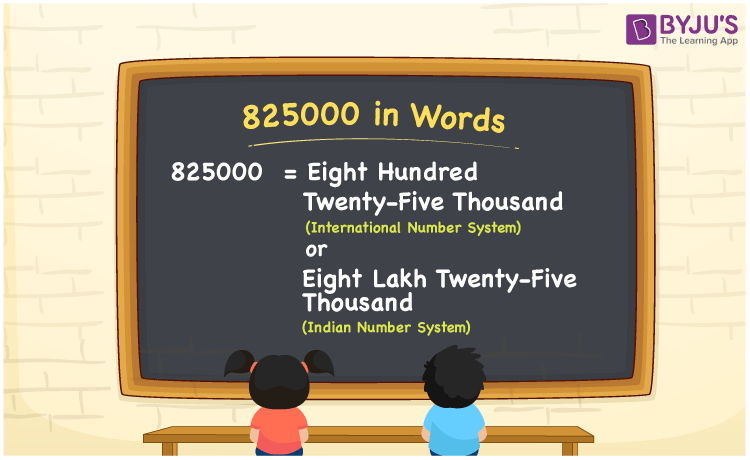# 825000 in Words

825000 in words is Eight hundred twenty-five thousand in the International number system and is Eight lakh twenty-five thousand in the Indian number system. As we know, 825000 is a six-digit number, we can express it in different ways considering International and Indian numeral systems. Let’s understand how to write the cardinal number 825000 in words using both International and Indian number systems, here in this article.

 825000 in words Eight hundred twenty-five thousand  (or) Eight lakh twenty-five thousand Eight hundred twenty-five thousand  (or) Eight lakh twenty-five thousand in Numbers 825000

## 825000 in English Words

We generally write numbers in words using the English alphabet. So, we spell 825000 in English as “Eight lakh twenty-five thousand or Eight hundred twenty-five thousand”.## How to Write 825000 in Words?

825000 in words – International number system

The below table is called the place value chart for 825000 with respect to the International number system.

 Hundred thousands Ten thousands Thousands Hundreds Tens Ones 8 2 5 0 0 0

Here, ones = 0, tens = 0, hundreds = 0, thousands = 5, ten thousands = 2, hundred thousands = 8

By expanding these digits, we get;

8 × 100 thousand + 2 × 10 thousand + 5 × thousand

= 800 thousand + 20 thousand + 5 thousand

= (800 + 20 + 5) thousand

= 825 thousand

Therefore, 825000 in words = 825 thousand = Eight hundred twenty-five thousand

825000 in words – Indian number system

Below is the place value chart for 825000 in the Indian system.

 Lakhs Ten thousands Thousands Hundreds Tens Ones 8 2 5 0 0 0

Here, ones = 0, tens = 0, hundreds = 0, thousands = 5, ten thousands = 2, lakhs = 8

These numbers can be expanded as follows.

= 8 × lakh + 2 × ten thousand + 5 × thousand + 0 × hundred + 0 × tens + 0 × ones

= Eight lakh + Twenty thousand + Five thousand

=Eight lakh twenty-five thousand

Therefore, 825000 in words = Eight lakh twenty-five thousand.

825000 is a natural number that precedes 825001 and succeeds 824999.

825000 in words – Eight lakh twenty-five thousand (or) Eight hundred twenty-five thousand

Is 825000 an odd number? – No

Is 825000 an even number? – Yes

Is 825000 a perfect square number? – No

Is 825000 a perfect cube number? – No

Is 825000 a prime number? – No

Is 825000 a composite number? – Yes

## Frequently Asked Questions on 825000 in Words

Q1

### How do you write 825000 in words?

We can write 825000 in words as Eight hundred twenty-five thousand or Eight lakh twenty-five thousand.
Q2

### How to write Rs. 825000 in words on a cheque?

On a cheque, we generally write Rs. 825000 in words as “Eight hundred twenty-five thousand rupees only or Eight lakh twenty-five thousand rupees only”.
Q3

### Write Eight lakh twenty-five thousand in numbers.

In the Indian number system, Eight lakh twenty-five thousand represents the number 825000.